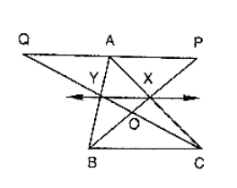# In figure, CD ∥ AE and CY ∥ BA.

Question:

In figure, CD ∥ AE and CY ∥ BA.

(i) Name a triangle equal in area of ΔCBX

(ii) Prove that ar(ΔZDE) = ar(ΔCZA)

(iii) Prove that ar(BCZY) = ar(ΔEDZ)Solution:

Since, triangle BCA and triangle BYA are on the same base BA and between same parallel s BA and CY.

Then ar(ΔBCA) = ar(ΔBYA)

⇒ ar(ΔCBX) + ar(ΔBXA) = ar(ΔBXA) + ar(ΔAXY)

⇒ ar(ΔCBX) = ar(ΔAXY) ⋅⋅⋅⋅ (1)

Since, triangles ACE and ADE are on the same base AE and between same parallels CD and AE

ar(ΔCZA) + ar(ΔAZE) = ar(ΔAZE) + ar(ΔDZE)

ar(ΔCZA) = ar(ΔDZE) ⋅⋅⋅ (2)

Adding ar(ΔCYG) on both sides , we get

⇒ ar(ΔCBX) + ar(ΔCYZ) = ar(ΔCAY) + ar(ΔCYZ)

⇒ ar(BCZY) = ar(ΔCZA) ⋅⋅⋅ (3)

Compare equation 2 and 3

⇒ ar(BCZY) = ar(ΔDZE)